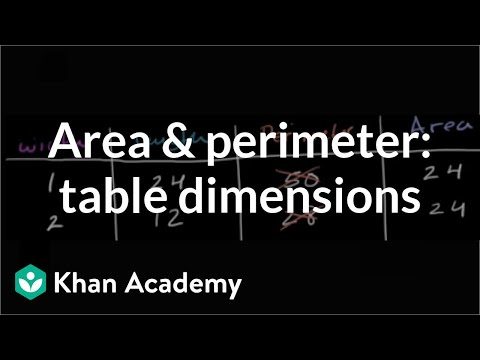Video

# Comparing areas and perimeters of rectangles (Full video)

Description: Sal compares the areas and perimeters of rectangles to a given rectangle. Created by Sal Khan. And we also know that this yellow rectangle has an area of 60 square units, whatever units we're measuring this 10 in. Well, to figure out perimeter, we would need to know the lengths of all the sides. Well, if the area is 60 square units, that means the length times the width is equal to 60, that 10 times this width right over here is going to be equal to 60.

### Other videos you might be interested in### Area & perimeter word problem: dog pen (Full video)